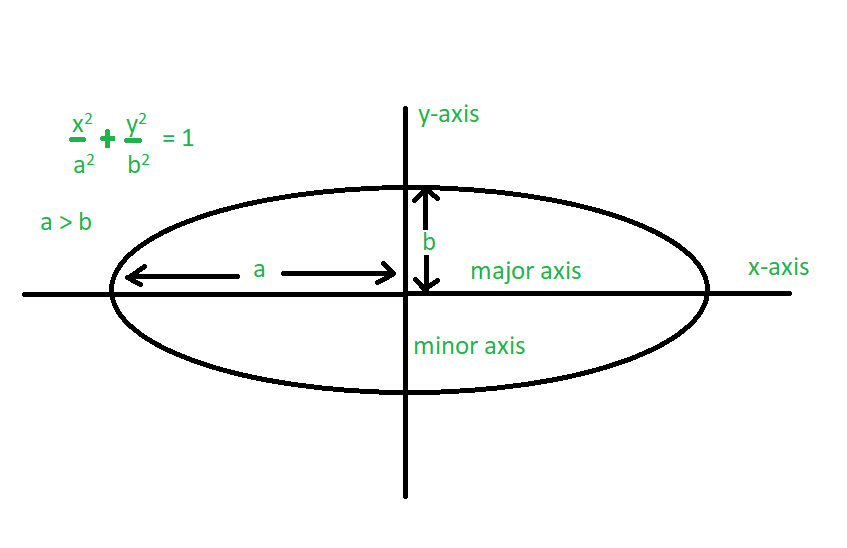Perimeter of an Ellipse

• Last Updated : 12 Jul, 2021

An ellipse is described as a curve on a plane that surrounds two focal points such that the sum of the distances to the two focal points is constant for every point on the curve. Ellipse has two types of axis – Major Axis and Minor Axis. The longest chord of the ellipse is the major axis. The perpendicular chord to the major axis is the minor axis which bisects the major axis at the center.
Given the lengths of minor and major axis of an ellipse, the task is to find the perimeter of the Ellipse.Examples:

Input: a = 3, b = 2
Output: 16.0109
Input: a = 9, b = 5
Output: 45.7191

Perimeter of an ellipse is

Perimeter : 2π * sqrt( (a2 + b2) / 2 )

Where a and b are semi-major axis and semi-minor axis respectively.
Below is the implementation of the above approach:

C++

 // C++ program to find perimeter of an Ellipse#include using namespace std; // Function to find the perimeter of an Ellipsevoid Perimeter(int a, int b){    float perimeter;     // Compute perimeter    perimeter = 2 * 3.14 * sqrt((a * a + b * b) / (2 * 1.0));     cout << perimeter;} // Driver codeint main(){    int a = 3, b = 2;     // Function call    Perimeter(a, b);     return 0;}

Java

 // Java program to find perimeter of// an Ellipse.import java.lang.Math;class GFG1{       // Function to find perimeter of an    // ellipse.    static void Perimeter( double a, double b)    {        double Perimeter;                   // formula to find the Perimeter        // of an Ellipse.        Perimeter = (double)2 * 3.14 * Math.sqrt((a * a + b * b) / (2 * 1.0)) ;                   // Display the result        System.out.println("Perimeter: " + Perimeter);    }           // Driver code    public static void main (String[] args)    {        double a = 3, b = 2;                   Perimeter(a , b);    }}

Python3

 # Python3 program to find perimeter# of an Ellipsefrom math import sqrt # Function to find the perimeter# of an Ellipsedef Perimeter(a, b):    perimeter = 0     # Compute perimeter    perimeter = (2 * 3.14 *                sqrt((a * a + b * b) /                           (2 * 1.0)));     print(perimeter) # Driver codea = 3b = 2 # Function callPerimeter(a, b) # This code is contributed# by Mohit Kumar

C#

 // C# program to find perimeter of// an Ellipse.using System;     class GFG1{         // Function to find perimeter of an    // ellipse.    static void Perimeter(double a, double b)    {        double Perimeter;                     // formula to find the Perimeter        // of an Ellipse.        Perimeter = (double)2 * 3.14 *                     Math.Sqrt((a * a + b * b) / (2 * 1.0));                     // Display the result        Console.WriteLine("Perimeter: " + Perimeter);    }             // Driver code    public static void Main (String[] args)    {        double a = 3, b = 2;                     Perimeter(a , b);    }} // This code is contributed by Princi Singh

Javascript



Output :

16.010921272681344

My Personal Notes arrow_drop_up